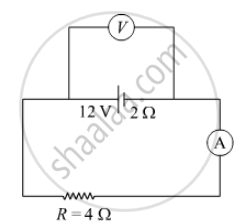Department of Pre-University Education, KarnatakaPUC Karnataka Science Class 12

# In the figure shown, an ammeter A and a resistor of 4 Ω are connected to the terminals of the source. The emf of the source is 12 V having an internal resistance of 2 Ω. Calculate the voltmeter and ammeter readings - Physics

In the figure shown, an ammeter A and a resistor of 4 Ω are connected to the terminals of the source. The emf of the source is 12 V having an internal resistance of 2 Ω. Calculate the voltmeter and ammeter readings.#### SolutionConsidering voltmeter and ammeter to be ideal. Then

I = 12/(4 + 2)

= 2 A

V = 12 − I × 2

= 12 − 2 × 2

=8 V

Concept: Electric Potential Difference
Is there an error in this question or solution?